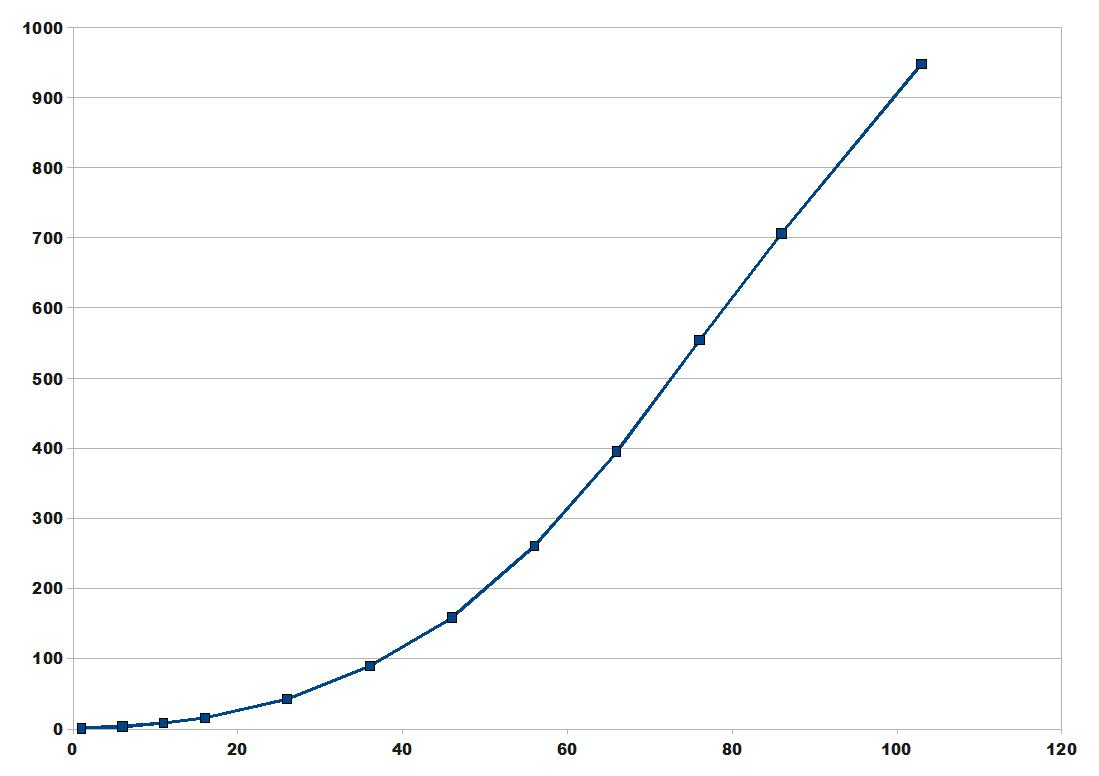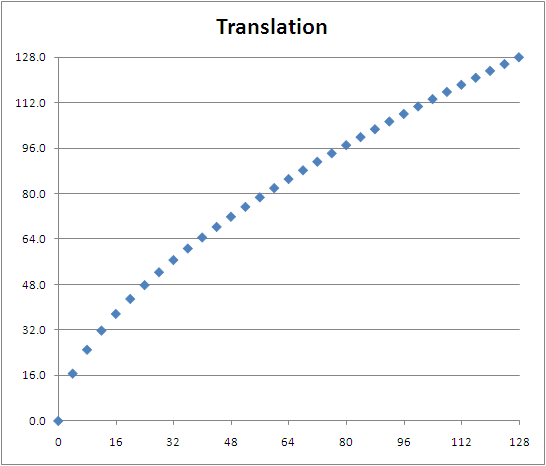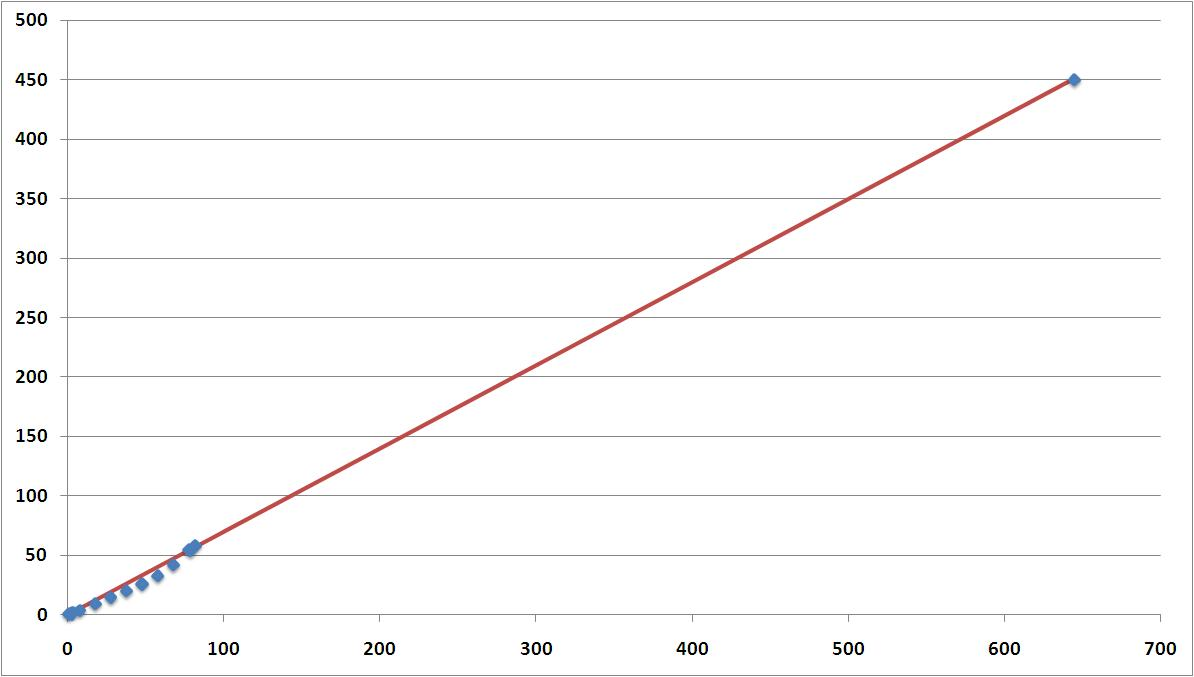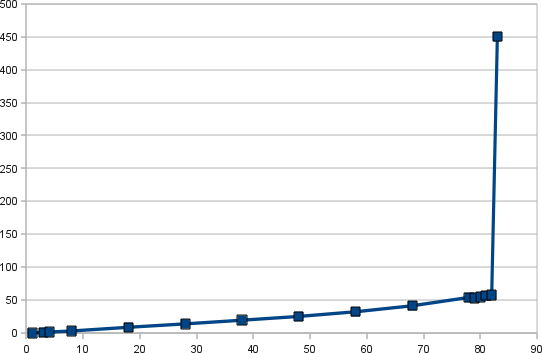## Subposition precision, 1:1 translation

This post explains a mechanism for the mouse to stick translation, that allows to reach a better precision and that is required to perform a 1:1 translation.

If you don’t want to read some math, then skip this post 🙂

All values are considered positive so as to simplify the explanation.

Each 10 ms, the sixaxis emulator processes all pending events.
All mouse motion events are merged and as a result, a single mouse event is processed.
This mouse event contains cumulated x and y deltas: (dx1, dy1).

This motion vector is then translated into a stick position according to the following formula:
`  (1) pos1 = dz1 + mul * pow(mv1, exp)`
pos1 = desired stick position (real)
dz1 = deadzone (real, function of dx1, dy1 and the deadzone shape)
mv1 = mouse value (integer), dx1 or dy1
mul = multiplier (real, constant)
exp = exponent (real, constant)

The result pos1 is a real. But the final stick position has to be an integer.
What emuclient was doing previously is rounding pos1 to the nearest integer.
This obviously results into a lower or higher speed than the desired one.
A much more precise solution to tackle this real to integer conversion is to truncate pos1, and compute a mouse vector remainder to be added next iteration.

The mouse event that corresponds to the applied stick position is: (dx2, dy2).
dx2 and dy2 are reals.
`  (2) pos2 = dz1 + mul * pow(mv2, exp)`
pos2 = applied stick position (truncate(pos1), integer)
dz1 = deadzone (same value, the angle is considered to be the same)
mv2 = mouse value (real), dx2 or dy2
mul = multiplier (real, constant)
exp = exponent (real, constant)

Note: norm(mv1) >= norm(mv2)
`  (3) vrem = mv1 - mv2`
vrem = mouse vector remainder
mv1 is known.
mv2 is computed from (2):
`  (4) mv2 = pow[(pos2 - dz1)/mul, 1/exp]`
mv2 can be replaced in (3):
`  (5) vrem = mv1 - pow[(pos2 - dz1)/mul, 1/exp]`
Conclusion:

• (5) gives the mouse vector remainder that has to be added the next iteration
• this emulates a subposition precision

Notes:

• this is useful with 8-bit precision for stick axes as the speed difference between two neighbor stick positions is high
• this is useless with 16-bit precision for stick axes

## What’s next?

Version 0.20 is bringing many new stuffs and I hope its quality is good. I was thinking to code more things for this release, but it would have even more delayed the release date. Issues 66, 68 and 70 (enhancements, not bugs) will probably be fixed in the next release.

There also are a few things I want to work on.

• Translation tables

I already studied the stick position to rotation speed relation some time ago (link). Measuring rotation speed for each stick position is quite painful, and I’m thinking to design a tool to automate it. I want this to work with a cheap video capture card (about 10\$).
Why do I want to study rotation speed? I want to add some translation tables (there will be one different translation table for each game) so that a 1:1 translation can be achieved. A 1:1 translation means that if you double your mouse speed, your rotation speed will double. This aims to produce a better PC-feel.

Following chart gives the rotation speed vs stick position measured for COD:MW2:It’s obviously not 1:1, especially for positions lower than 50.

Following table (values do not correspond to the above chart) shows how to perform a 1:1 translation:

1:1 translation
position speed 1:1 speed 1:1 position
1 0 8 7
32 91 140 57
64 295 480 85
96 589 720 108
128 960 960 128

All stick positions are required. Speed unit is Â°/s.

First column is the stick position.
Second column is the measured rotation speed.
Third column is the speed that we would expect with a 1:1 position to rotation speed translation (position*max speed/max position).
Fourth column is the translation of the position to perform a 1:1 translation. Look for the expected speed in the 2nd column (closest value) and look what position it is in the first column, this gives the 1:1 position.

The translation looks like this:This position to position translation may introduce a loss of precision. It probably would be better to have a direct speed to position translation.
• Better precision for the right analog stick

8-bit precision allows 256 stick positions. 10-bit precision allows 1024 stick positions. More positions give a finer control. As a comparison, a high-end mouse reports x and y values with a 16 bit precision.

The official sixaxis page tells that analog sticks and l2/r2 buttons have a 10-bit precision. This is wrong: stick and button positions are reported with a 8-bit only precision. Only motion sensing controls have a 10-bit precision. This is lame because many people are saying that the Xbox360 pad is technically lower than the Sixaxis (8-bit precision vs 10-bit precision), which is absolutely wrong.

We can’t change the precision of the sixaxis emulator, but it is probably possible to build a usb gamepad interface that has a precision higher than 8-bit for the right stick.

## Mouse mapping improvement – 2

Last mouse mapping improvement was about tweaking the dead zone shape, and was proposed by a reader of this blog. Another reader suggested me to change the way the mouse motion is processed.

The sixaxis emulator currently approximates the velocity from the mouse report average over 10ms (=the sixaxis report period). For ex, if it receives N mouse reports, it assumes a constant 10/N ms period.

Example 1:

``````
t0-1ms report x = some value
t0 all mouse report processed, a report is sent to the ps3, x = 0
t0 report x = +10
t0+1ms report x = +8
t0+2ms report x = +6
t0+3ms report x = +4
t0+4ms report x = +2
... no other report
t0+10ms all mouse report processed, a report is sent to the ps3``````

``````
5 reports, report period is approximated to 2ms.
Mouse report average: (10+8+6+4+2)/5 = 6 units/ms``````

Well, this is overestimated.

``````
With a simple accumulation + division:
Mouse report average: (10+8+6+4+2)/10 = 3 units/ms``````

For this example, the computed velocity is 2x the real one o_O

This is not harmful if the computed velocity is always 2x the real one.
But let’s take another example.

Example 2:

``````
t0-1ms  report x = some value
t0      all mouse report processed, a report is sent to the ps3, x = 0
t0      report x = +10
t0+1ms  report x = +8
t0+2ms  report x = +6
...     no other report
t0+10ms all mouse report processed, a report is sent to the ps3``````

``````
3 reports, report period is approximated to 3/10ms.
Mouse report average: (10+8+6)/3 = 8 units/ms``````

``````
With a simple accumulation + division:
Mouse report average: (10+8+6)/10 = 2.4 units/ms``````

For this example, the computed velocity is 3.33x the real one…

The previous computation was introducing a non constant multiplier 🙁

## Mouse mapping improvement

Each polling period (=10ms=sixaxis refresh rate), emuclient processes all queued events.
This means that depending on the mouse refresh rate, emuclient may process one or several mouse motion events.

Examples:

• (rate=100Hz) => 1 event max
• (rate=500Hz) => 5 events max
• (rate=1000Hz) => 10 events max

In a single polling period, emuclient may receive several events, but only performs a single sixaxis status refresh. Therefore, mouse motion events have to be merged into a single one that is the average mouse motion event. This allows to reach a better precision since no event is ignored.

The average mouse motion event is translated to a sixaxis axis thanks to the following formula:

res=sign(val)*mul*pow(abs(val),exp)

I usually use exp=1 so that the simplified formula is:

res=mul*val

The dead zone has also to be removed:

if res>0: res=res+dz
if res<0: res=res-dz

Different multipliers, exponent and dead zones may be set for x and y mouse motions.
This calculation supposes the dead zone is rectangular.

Following to the suggestion of a reader, I decided to test a different dead zone shape.

if x!=0 and y!=0:
dz_x=dz*cos(atan(abs(y/x)))
dz_y=dz*sin(atan(abs(y/x)))
else:
dz_x=dz
dz_y=dz

I also improved the result precision by storing each intermediate result as a double (and not an integer). Only the final result is converted to an integer.

I built a test package for people that want to try these new things.
Just remember to set the same value for both x and y dead zones.
Let me know the results of your tests!

## Mouse to joystick translation – Call Of Duty Modern Warfare 2

COD MW2 has a totally different behavior regarding the position to rotation speed relation.

Following chart gives the rotation speed (degrees per second) given the absolute position of z.
The joystick sensibility is set to the highest position.The rotation speed is continuous and goes from low to very high values, so that the mouse to joystick translation is very easy to do: a simple x3 multiplier is sufficient.

The acceleration looks like the mouse acceleration that is performed under windows xp (described there).

## Mouse to joystick translation – FARCRY2 – part 2

It was explained in part 1 that absolute positions 1 to 82 give a linear rotation speed (from 0 to about 50Â°/s), and position 83 a high rotation speed (450Â°/s for z, 200Â°/s for rz).

Following chart gives the rotation speed for a given virtual position of z that goes from 0 to 645. That gives a linear speed from 0 to 450Â°/s. Virtual positions from 83 to 644 have to be created.Blue dots are speeds that are achieved by real positions with a standard ps3 controller.
Red line is obtained with a simple linear regression.

Each mouse packet applies a rotation speed over a time that is the inverse of the mouse frequency (for ex 100Hz => 10ms).

A rotation speed for a virtual position between 83 and 644 may be generated “in average”.

For example, if a speed of 450Â°/s is applied (virtual position 645=real position 83) over 5ms, and 0Â°/s is applied (real position 0) over 5ms, the average speed that is obtained over 10ms is 225Â°/s (virtual position 321).

0+83 makes crappy movements, that technique actually works great with 82+83

I realized afterward that it is similar to PWM.

## Mouse to joystick translation – FARCRY2 – part 1

(x,y) speed of the mouse has to be mapped to a (z,rz) rotation speed.

z and rz are in the range [0..255] with [83..173] as dead zone (128 is the center).

Following chart gives the rotation speed (degrees per second) given the absolute position of z.

This probably may change with the game (values are for Farcry).This is quite linear except for the max position (83). The max speed that is in the linear area (position 82, about 50Â°/s) is not fast enough to only use the linear area. The speed of the max position is so fast (about 450Â°/s) that it shouldn’t be used over a long time.

There is the same behavior for rz, except that the speed of the max position is about 200Â°/s).

A heuristic has to be found to trigger the max position use.